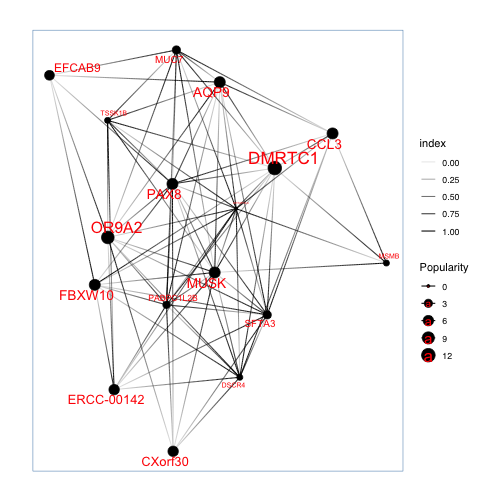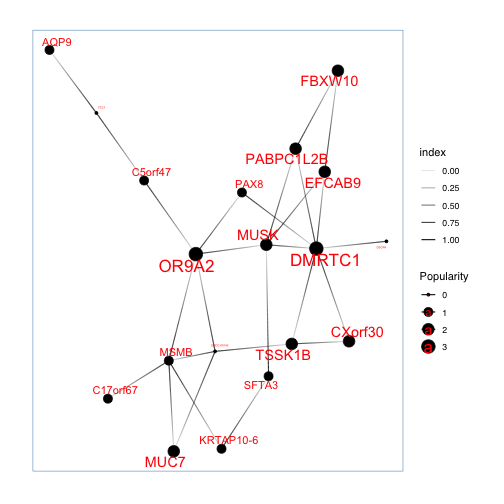pGRN package pre-loaded with pGRNDB data with both of expression matrix and pseudotime information. You can simply load this data by calling pGRNDB.

``````# loading pGRN package
library(pGRN)

example_data <- pGRNDB
names(example_data)
``````
``````##  "expression" "ptime"
``````
``````expression_matrix <- example_data[["expression"]]
pseudotime_list <- example_data[["ptime"]]\$PseudoTime
``````

## Call pGRN

Currently, two methods “DTW” and “granger” were available for pGRN network construction.

``````# try DTW method
nets_dtw <- pGRN(expression_matrix,pseudotime_list, method= "DTW",quantile_cutoff=50)

# try granger method
nets_gg <- pGRN(expression_matrix,pseudotime_list, method= "granger")
``````
``````## Time relapsed for granger test: 1.6192889213562 seconds!
``````

## plot the network

pGRN provides methods for visualize the network either stationary or interactively.

``````# plot network stationarily
plot_network(nets_dtw[])
````````````plot_network(nets_gg[])
````````````# plot network interactively
#plot_network_i(nets_dtw[])
#plot_network_i(nets_gg[])
``````